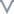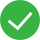# 数值函数

### 数值函数的作用

#### 示例

``````ABS([利润差异])
``````

### 平台中的数值函数

ABS - 返回给定数字的绝对值。

ACOS - 返回给定数字的反余弦。结果以弧度表示。

ASIN - 返回给定数字的反正弦。结果以弧度表示。

ATAN - 返回给定数字的反正切。结果以弧度表示。

ATAN2 - 返回两个给定数字（x 和 y）的反正切。结果以弧度表示。

CEILING - 将数字舍入为值相等或更大的最近整数。

COS - 返回角度的余弦。以弧度为单位指定角度。

COT - 返回角度的余切。以弧度为单位指定角度。

DEGREES - 将以弧度表示的给定数字转换为度数。

DIV - 返回将整数 1 除以整数 2 的除法运算的整数部分。

EXP - 返回 e 的给定数字次幂。

FLOOR - 将数字舍入为值相等或更小的最近整数。

LN - 返回数字的自然对数。

LOG - 返回数字以给定底数为底的对数。

MAX - 返回两个参数（必须为相同类型）中的较大值。

MIN - 返回两个参数（必须为相同类型）中的较小值。

PI - 返回数字常量 pi：3.14159。

ROUND - 将数字舍入为指定位数。

SIGN - 返回数字的符号。

SIN - 返回角度的正弦。以弧度为单位指定角度。

SQRT - 返回数字的平方根。

SQUARE - 返回数字的平方。

TAN - 返回角度的正切。以弧度为单位指定角度。

ZN - 如果表达式不为 Null，则返回该表达式，否则返回零。使用此函数可使用零值而不是 Null值。

ABS(数值)

CEIL(数值)

CEIL(3.1415) = 4

## 下取整：FLOOR(数值)

FLOOR(数值)

#### 示例

FLOOR(200.7) = 200，FLOOR(-1.5) = -2

PI(数值)

PI() = 3.1415926

EXP(数值)

EXP(3) = e^3

## DEGRESS(数值)

DEGRESS(数值)

#### 示例

DEGREES(PI( )/4) = 45

## SIN(数值)

SIN(数值)

#### 示例

SIN(PI()/4) = 0.707106

## COS(数值)

COS(数值)

#### 示例

COS(PI( ) /4) = 0.707106

## TAN(数值)

TAN(数值)

#### 示例

TAN(PI ( )/4) = 1

## COT(数值)

COT(数值)

#### 示例

COT(PI ( )/4) = 1

## ASIN(数值)

ASIN(数值) 返回数字的反正弦。结果以弧度表示

#### 示例

ASIN(1) = 1.570796

## ACOS(数值)

ACOS(数值) 返回数字的反正弦。结果以弧度表示

#### 示例

ACOS(-1) = 3.1415926

## ATAN(数值)

ATAN(数值) 返回数字的反正切。结果以弧度表示

#### 示例

ATAN(180) = 1.56524

## ATAN2(数值1,数值2)

ATAN2(数值1,数值2) 返回两个给定数字的反正切，结果以弧度表示。

#### 示例

ATAN2(2，1) = 1.107148

## POWER(底数, 指数)

POWER(底数, 指数) 返回底数的指数次方。

#### 示例

POWER(2, 3) = 2 2 2 = 8

## LOG(底数, 真数)

LOG(底数, 真数) 返回真数以底数为底的对数。

LOG(2, 16) = 4

## ROUND(表达式,小数位数)

ROUND(表达式,小数位数) 将数字四舍五入为最接近的整数或指定小数位数。

#### 示例

ROUND(3.1415,2)=3.14根本没帮助文档较差文档一般文档不错文档很好

#### 更多建议

0/200